# Class 9 RD Sharma Solutions – Chapter 8 Introduction to Lines and Angles- Exercise 8.3

• Last Updated : 27 Dec, 2021

### Question 1. In the below fig, lines l1, and l2 intersect at O, forming angles as shown in the figure. If x = 45°. Find the values of x, y, z, and u.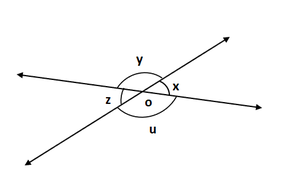Solution:

Given that X = 45°

Find: the value of Y, Z, and u

z = x = 45° [Vertically opposite angles are equal]

z + u = 180° [z and u are angles that are a linear pair]

z = 180° – u

u = 180° – x

u = 180° – 45°

u = 135°

x + y = 180° [x and y angles are a linear pair]

y = 180° – x

y =180° – 45°

y = 135°

Hence, x = 45°, y = 135°, z = 135° and u = 45°

Question 2. In the below fig. three coplanar lines intersect at a point O, forming angles as shown in the figure. Find the values of x, y, z and u.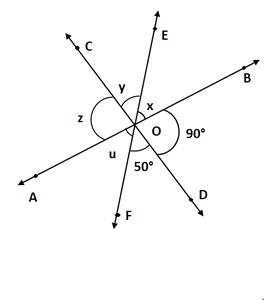Solution:

From the given figure

∠SOD = z = 90°     [Vertically opposite angles are equal]

∠DOF = y = 50°

Now, x + y + z = 180°   [Linear pair of angles]

Now put the value of z and y

90° + 50° + x = 180°

x = 180° – 140°

x = 40°

So, x = 40°, y = 50°, z = 90°, u = 40°

### Question 3. In the given fig, find the values of x, y, and z.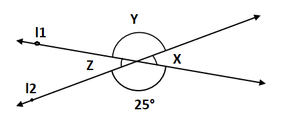Solution:

From the given figure

y = 25°    [Vertically opposite angles are equal]

Now ∠x +∠y = 180°    [Linear pair of angles]

x = 180° – 25°

x = 155°

Also,

z = x = 155°    [Vertically opposite angles]

y = 25°

z = 155°

So, x = 155°, y = 25°, z = 155°

### Question 4. In the below fig. find the value of x?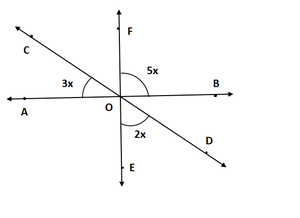Solution:

From the figure

AOE = BOF = 5x    [Vertically opposite angles are equal]

∠COA + ∠AOE + ∠EOD = 180°   [Linear pair angles]

3x + 5x + 2x = 180°

10x = 180°

x = 180°/10

x = 18°

So, the value of x = 18°

### Question 5. Prove that bisectors of a pair of vertically opposite angles are in the same straight line.

Solution: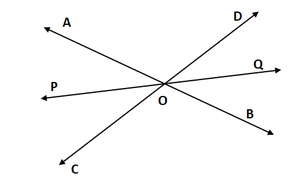From the figure

Lines AB and CD intersect at point O, such that

∠AOC = ∠BOD    [vertically opposite angles are equal] …(1)

Also, OP is the bisector of AOC and OQ is the bisector of BOD

To Prove: POQ is a straight line.

∠AOP = ∠COP    [OP is the bisector of ∠AOC]…(2)

∠BOQ = ∠QOD   [OQ is the bisector of ∠BOD]…(3)

Now,

∠AOC + ∠BOD + ∠AOP + ∠COP + ∠BOQ + ∠QOD = 360°        [sum of all angles around a point is 360°]

∠BOQ + ∠QOD + ∠DOA + ∠AOP + ∠POC + ∠COB = 360°

2∠QOD + 2∠DOA + 2∠AOP = 360° (from eq(1), (2) and (3))

∠QOD + ∠DOA + ∠AOP = 180°

POQ = 180°

Hence proved

### Question 6. If two straight lines intersect each other, prove that the ray opposite to the bisector of one of the angles thus formed bisects the vertically opposite angle.

Solution: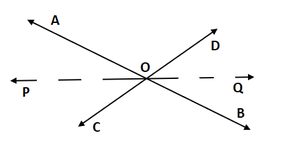Let us considered AB and CD intersect at a point O

Now draw the bisector OP of AOC

OP = POC … (i)

Let extend OP to Q.

Show that, OQ bisects BOD

Let us considered that OQ bisects BOD,

Prove that POQ is a line.

As we know that,

AOC = DOB  …. (ii)  [vertically opposite angles.]

AOP = BOQ   [vertically opposite angles.]

Similarly, POC = DOQ

AOP + AOD + DOQ + POC + BOC + BOQ = 360°  [sum of all angles around a point is 360 degrees ]

2AOP + AOD + 2D0Q + BOC = 360°

2AOP + 2AOD + 2DOQ = 360°

2(AOP + AOD + DOQ) = 360°

AOP + AOD +DOQ = 360°/2

AOP + AOD + DOQ = I80°

Thus, POQ is a straight line.

Hence proved

### Question 7. If one of the four angles formed by two intersecting lines is a right angle. Then show that each of the four angles is a right angle.

Solution: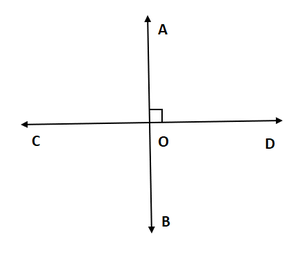According to question

AB and CD intersecting at O, such that ∠BOC = 90°, ∠AOC = 90 °∠AOD = 90° and ∠BOD = 90°

Given:∠BOC = 90°

∠BOC = ∠AOD = 90°    [Vertically opposite angles are equal]

∠AOC + ∠BOC = 180°    [Angles in linear pair]

∠AOC + 90°  = 180°  [Angles in linear pair]

∠AOC = 90°∠AOC = ∠BOD = 90°    [Vertically opposite angles]

Hence, ∠AOC = ∠BOC = ∠BOD = ∠AOD = 90°

### Question 8. In the below fig. rays AB and CD intersect at O.

(i) Determine y when x = 60°

(ii) Determine x when y = 40°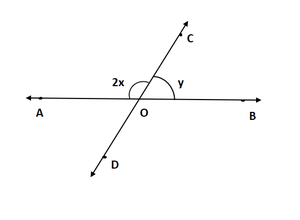Solution:

(i) Given that x = 60°

∠AOC + ∠BOC = 180°   [linear pair of angles]

⟹ 2x + y = 180°

⟹ 2(60°) + y = 180° [since x = 60°]

⟹ y = 60°

Hence, the value of y = 60° when x = 60°

(ii) Given y = 40°

∠AOC + ∠BOC = 180°   [linear pair of angles]

⟹ 2x + y = 180°

⟹ 2x + 40° = 180°  [since x = 40°]

⟹ 2x =180° – 140°

⟹ 2x = 140°

⟹ x = 70°

Hence, the value of x = 70° when x = 40°

### Question 9. In the below fig. lines AB. CD and EF intersect at O. Find the measures of ∠AOC, ∠COF, ∠DOE, and ∠BOF.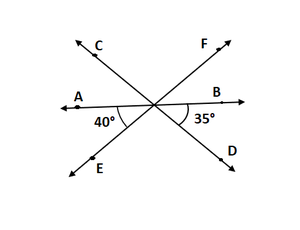Solution:

From the figure

∠AOE + ∠EOB = 180°   [linear pair of angles]

∠AOE + ∠DOE + ∠BOD = 180°  [linear pair of angles]

⟹ ∠DOE = 180° – 40° – 35° = 105°

∠DOE = ∠COF = 105°    [Vertically opposite angles are equal]

Now, ∠AOE + ∠AOF = 180°   [Angles in Linear pair]

∠AOE + ∠AOC + ∠COF = 180°

⟹ 40° + ∠AOC +105° = 180°

⟹ ∠AOC = 180° – 145°

⟹ ∠AOC = 35°

Also, ∠BOF = ∠AOE = 40° [Vertically opposite angles are equal]

Hence, the value of ∠AOC = 35°, ∠COF = 105°, ∠DOE = 105°, and ∠BOF = 40°

### Question 10. AB, CD, and EF are three concurrent lines passing through the point O such that OF bisects BOD.  If ∠BOF = 35. Find ∠BOC and ∠AOD.

Solution: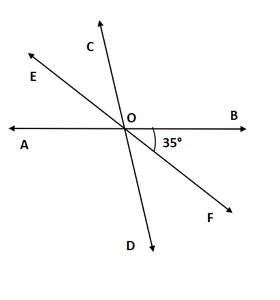Given that OF bisects ∠BOD

∠BOF = 35°

We have to find ∠BOC and ∠AOD

∠BOD = 2 ∠BOF = 70°            [since OF bisects ∠BOD]

∠BOD = ∠AOC = 70°                 [ vertically opposite angles]

Now,

∠BOC + ∠AOC = 180°

∠BOC + 70° = 180°

∠BOC = 110°

∠AOD = ∠BOC = 110°             [Vertically opposite angles]

Hence, the value of ∠BOC = 110° and ∠AOD = 110°

### Question 11. In the below figure, lines AB and CD intersect at O. If ∠AOC + ∠BOE = 70° and ∠BOD = 40°, find ∠BOE and reflex ∠COE?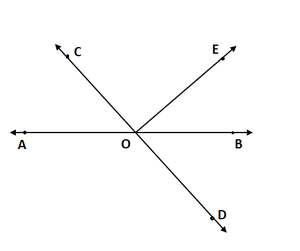Solution:

Given: AOC + BOE = 70° and BOD = 40°

We have to find ∠BOE and reflex ∠COE

BOD = AOC = 40°  [vertically opposite angles]

∠AOC + ∠BOE = 70°  [given]

⟹ 40° + ∠BOF = 70°

⟹ ∠BOF = 70° – 40°

⟹ ∠BOE = 30°

⟹ AOC + COF + BOE = 180°  [Angles in linear pair]

⟹ COE = 180° – 30° – 40°

⟹ COE = 110°

Reflex ∠COE = 360° – 110° = 250°
Hence, the value of ∠BOE = 30° and ∠COE =250°

### Question 12. Which of the following statements are true (T) and which are false (F)?

(i) Angles forming a linear pair are supplementary.

(ii) If two adjacent angles are equal and then each angle measures 90°

(iii) Angles forming a linear pair can both acute angles.

(iv) If angles forming a linear pair are equal, then each of the angles have a measure of 90°

Solution:

(i) True

(ii) False

(iii) False

(iv) true

### Question 13. Fill in Inc blanks so as to make the following statements true:

(i) If one angle of a linear pair is acute then its other angle will be______

(ii) A ray stands on a line, then the sum of the two adjacent angles so formed is ______

(iii) If the sum of two adjacent angles is 180°, then the ______ arms of the two angles are opposite rays.

Solution:

(i) Obtuse angle

(ii) 180°

(iii) Uncommon

My Personal Notes arrow_drop_up